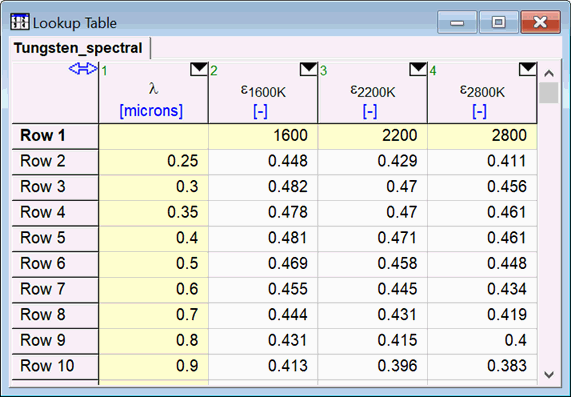Contents - Index

INTERPOLATE2DM

The INTERPOLATE2DM function provides two-dimensional linear interpolation for data in a Lookup table or Lookup file that are arranged in a row and column matrix format.  Note that the INTERPOLATE2D function also provides two-dimensional interpolation, but for this function, the data must be arranged in three columns and a gridding method is applied to the data.

The INTERPOLATE2DM function has the following format.

INTERPOLATE2DM('Table Name', X, Y)

'Table Name' is a string constant or string variable  that provides the name of the Lookup table in the Lookup Window or the name of an existing Lookup file stored on disk. The string constant must be enclosed within single quote marks.  Lookup table names appear on the tabs at the top of the Lookup Table Window.   If the name of a disk file is supplied, it must be the name of an existing Lookup file having either the binary (.LKT) or ASCII (.TXT, .CSV, or .DAT) Lookup file Formats.

X is the value of the independent variable whose values appear in the first row of the table.   The columns used to determine the interpolated value will be identified by this value.

Y is the value of the independent variable whose values appear in the first column of the table.   The rows used to determine the interpolated value will be identified by this value.

The capabilities of the INTERPOLATE2DM function are best illustrated by example.  Shown below is a Lookup table containing the emittance of tungsten as a function of temperature and wavelength.  Note the temperature values are provided in the first row and the wavelength values are provided in the first column.   The remaining (unshaded) cells contain emittance values at the specified temperature and wavelength.  The following example will estimate the emittance at 2000 K and 0.55 microns.

T=2000 [K]

lambda=0.55 [µm]

Epsilon=INTERPOLATE2DM('Tungsten', T, lambda)The INTERPOLATED2DM function is actually a library routine (located in the USERLIB\EES_System folder) rather than a built-in function.  It uses the LookupCol1, LookupRow1, and Lookup functions.

See also:

Lookup

Interpolate

Interpolate2D